## Standard Free Energy Changes

The standard Gibbs Free Energy is calculated using the free energy of formation of each component of a reaction at standard pressure.

### Learning Objectives

Calculate the change in standard free energy for a particular reaction.

### Key Takeaways

#### Key Points

• The standard free energy of a substance represents the free energy change associated with the formation of the substance from the elements in their most stable forms as they exist under standard conditions.
• Combine the standard enthalpy of formation and the standard entropy of a substance to get its standard free energy of formation.
• $\Delta \text{G}^\circ (\text{rxn}) = \Sigma \Delta {\text{G}}_{\text{f}}^\circ (\text{products}) - \Sigma \Delta {\text{G}}_{\text{f}}^\circ (\text{reactants})$ can be used to determine standard free energy change of a reaction.

#### Key Terms

• enthalpy: In thermodynamics, a measure of the heat content of a chemical or physical system.
• entropy: A thermodynamic property that is the measure of a system’s thermal energy per unit temperature that is unavailable for doing useful work.
• free energy of formation: The change of free energy that accompanies the formation of 1 mole of a substance in its standard state from its constituent elements in their standard states.

### Reviewing Standard States

The concept of standard states is especially important in the case of free energy, so take a moment to review it. For most practical purposes, the following definitions of standard states are acceptable:

• Gases: 1 atmosphere partial pressure.
• Pure liquids: the liquid under a total pressure of 1 atm.
• Solutes: an effective concentration of 1 Molar.
• Solids: the pure solid under 1 atm pressure.

Note also that there is actually no “standard temperature “, but because most thermodynamics tables list values for 298.15 K (25° C), this temperature is usually implied. These same definitions apply to standard enthalpies and internal energies. Don’t confuse these thermodynamic standard states with the “standard temperature and pressure” (STP) widely employed in gas law calculations.

### Calculating Gibbs Free Energy

In order to make use of Gibbs energies to predict chemical changes, it is necessary to know the free energies of the individual components of the reaction. To accomplish this, combine the standard enthalpy and the standard entropy of a substance to get the standard free energy of a reaction:

$\Delta {\text{G}}^\circ= \Delta {\text{H}}^\circ-\text{T}\Delta {\text{S}}^\circ$

Recall that the symbol ° refers to the standard state of a substance measured under the conditions of 1 atm pressure or an effective concentration of 1 Molar and a temperature of 298K. The other factor to keep in mind is that enthalpy values are normally given in $\frac{\text{kJ}}{\text{mole}}$ while entropy values are given in $\frac{\text{J}}{\text{K}\times \text{mole}}$. The energy units will need to be the same in order to solve the equation properly.

The standard Gibbs free energy of the reaction can also be determined according to:

$\Delta \text{G}^\circ (\text{rxn})= \Sigma \Delta {\text{G}}_{\text{f}}^\circ(\text{products})-\Sigma \Delta {\text{G}}_{\text{f}}^\circ(\text{reactants})$

As with standard heats of formation, the standard free energy of a substance represents the free energy change associated with the formation of the substance from the elements in their most stable forms as they exist under the standard conditions of 1 atm pressure and 298K. Standard Gibbs free energies of formation are normally found directly from tables. Once the values for all the reactants and products are known, the standard Gibbs free energy change for the reaction can be found. Most tables of thermodynamic values list ΔGf°’s for common substances. They can, of course, always be found from values of $\Delta {\text{H}}_{\text{f}}^\circ$ and $\Delta {\text{S}}_{\text{f}}^\circ$.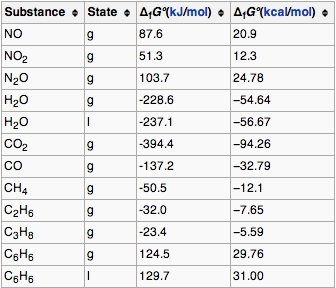Gibbs Energy of Formation: The standard Gibbs free energy of formation of a compound is the change of Gibbs free energy that accompanies the formation of 1 mole of that substance from its component elements, at their standard states.

Example Problem: Calculate the $\Delta \text{G}^0_{\text{rxn}}$ for the following equation using the values in the table:

$\text{CO}_{(\text{g})} + \frac {1}{2} \text{O}_{2(\text{g})} \rightarrow \text{CO}_{2(\text{g})}$

$\Delta \text{G}^\circ (\text{rxn})= \Sigma \Delta {\text{G}}_{\text{f}}^\circ(\text{products})-\Sigma \Delta {\text{G}}_{\text{f}}^\circ(\text{reactants})$

*Remember that substances in elemental form (such as O2) have $\Delta \text{G}^\text{o}_\text{f}$ values equal to zero. It is also important to remember that the table provides per mole values. If the balanced equation calls for more than one mole the $\Delta \text{G}^\text{o}_\text{f}$ must be multiplied.

$\Delta \text{G}^\circ (\text{rxn})= (-394.4\ \text{kJ}) -(-137.2\ \text{kJ} + 0\ \text{kJ})$

$\Delta \text{G}^\circ (\text{rxn})= -257.2\ \text{kJ}$

## Free Energy Changes in Chemical Reactions

ΔG determines the direction and extent of chemical change.

### Learning Objectives

Recall the possible free energy changes for chemical reactions.

### Key Takeaways

#### Key Points

• If the free energy of the reactants is greater than that of the products, the entropy of the world will increase when the reaction takes place as written, and so the reaction will tend to take place spontaneously.
• If the free energy of the products exceeds that of the reactants, then the reaction will not take place.
• An important consequence of the one-way downward path of the free energy is that once it reaches its minimum possible value, net change comes to a halt.
• In a spontaneous change, Gibbs energy always decreases and never increases.

#### Key Terms

• spontaneous change: A spontaneous process is the time-evolution of a system in which it releases free energy (usually as heat) and moves to a lower, more thermodynamically stable energy state.

### The Direction and Extent of Chemical Change

ΔG determines the direction and extent of chemical change. Remember that ΔG is meaningful only for changes in which the temperature and pressure remain constant. These are the conditions under which most reactions are carried out in the laboratory. The system is usually open to the atmosphere (constant pressure) and the process is started and ended at room temperature (after any heat that has been added or which was liberated by the reaction has dissipated.)

The importance of the Gibbs function can hardly be over-stated: it determines whether a given chemical change is thermodynamically possible. Thus, if the free energy of the reactants is greater than that of the products, the entropy of the world will increase and the reaction takes place spontaneously. Conversely, if the free energy of the products exceeds that of the reactants, the reaction will not take place.

In a spontaneous change, Gibbs energy always decreases and never increases. This of course reflects the fact that the entropy of the world behaves in the exact opposite way (owing to the negative sign in the TΔS term). Here is an example:

${\text{H}}_{2}\text{O}(\text {liquid}) \rightarrow {\text{H}}_{2}\text{O} (\text{ice})$

Water below zero degrees Celsius undergoes a decrease in its entropy, but the heat released into the surroundings more than compensates for this so the entropy of the world increases, the free energy of the H2O diminishes, and the process proceeds spontaneously.

An important consequence of the one-way downward path of the free energy is that once it reaches its minimum possible value, net change comes to a halt. This, of course, represents the state of chemical equilibrium. These relations are summarized as follows:

• $\Delta \text{G} < 0$: The reaction will occur spontaneously to the right.
• $\Delta \text{G} > 0$: The reaction will occur spontaneously to the left.
• $\Delta \text{G} = 0$: The reaction is at equilibrium and will not proceed in either direction.

### Conditions for Spontaneous Change

Recall the condition for spontaneous change:

ΔG = ΔH – TΔS < 0

where ΔG = change in Gibbs free energy, ΔH = change in enthalpy, T = absolute temperature, and ΔS = change in entropy

It is apparent that the temperature dependence of ΔG depends almost entirely on the entropy change associated with the process. (it is appropriate to say “almost” because the values of ΔH and ΔS are themselves slightly temperature dependent; both gradually increase with temperature). In particular, notice that in the above equation the sign of the entropy change determines whether the reaction becomes more or less spontaneous as the temperature is raised.

For any given reaction, the sign of ΔH can also be positive or negative. This means that there are four possibilities for the influence that temperature can have on the spontaneity of a process:

### Case 1: ΔH < 0 and ΔS > 0

Under these conditions, both the ΔH and TΔS terms will be negative, so ΔG will be negative regardless of the temperature. An exothermic reaction whose entropy increases will be spontaneous at all temperatures.

### Case 2: ΔH < 0 and ΔS < 0

If the reaction is sufficiently exothermic it can force ΔG to be negative only at temperatures below which |TΔS| < |ΔH|. This means that there is a temperature defined by $\text{T} = \frac{\Delta \text{H}}{\Delta \text{S}}$ at which the reaction is at equilibrium; the reaction will only proceed spontaneously below this temperature. The freezing of a liquid or the condensation of a gas are the most common examples of this condition.

### Case 3: ΔH > 0 and ΔS > 0

This is the reverse of the previous case; the entropy increase must overcome the handicap of an endothermic process so that TΔS > ΔH. Since the effect of the temperature is to “magnify” the influence of a positive ΔS, the process will be spontaneous at temperatures above $\text{T} = \frac{\Delta \text{H}}{\Delta \text{S}}$. (Think of melting and boiling. )

### Case 4: ΔH > 0 and ΔS < 0

With both ΔH and ΔS working against it, this kind of process will not proceed spontaneously at any temperature. Substance A always has a greater number of accessible energy states, and is therefore always the preferred form.

## Free Energy Changes for Nonstandard States

A single reaction can have an infinite number of ΔG values, reflecting the infinite possible compositions between reactants and products.

### Learning Objectives

Differentiate between free changes for standard and nonstandard states.

### Key Takeaways

#### Key Points

• ΔG° and ΔGf° refer to single, specific chemical changes in which all components ( reactants and products ) are in their standard states.
• The physical meaning of ΔG is that it tells us how far the free energy of the system has changed from G° of the pure reactants.
• When ΔG reaches its minimum value, the composition of the system is at its equilibrium value.

#### Key Terms

• free energy: The difference between the internal energy of a system and the product of its entropy and absolute temperature.
• equilibrium: The state of a reaction in which the rates of the forward and reverse reactions are the same.

The apparent similarity of the terms $\Delta \text{G}$, $\Delta \text{G}^{\circ}$ and $\Delta \text{G}_\text{f}^{\circ}$ makes it easy to overlook the distinctions between them; the similarity is a major source of confusion. The most important thing to remember is that the terms $\Delta \text{G}^{\circ}$and $\Delta \text{G}_\text{f}^{\circ}$ refer to single, specific chemical changes in which all components (reactants and products) are in their standard states.

### Plotting

The distinction is nicely illustrated in this diagram, in which $\Delta \text{G}$ is plotted on a vertical axis for two hypothetical reactions having opposite signs of $\Delta \text{G}^{\circ}$. The horizontal axis schematically expresses the relative concentrations of reactants and products at any point of the process. Note that the origin corresponds to the composition at which half of the reactants have been converted into products.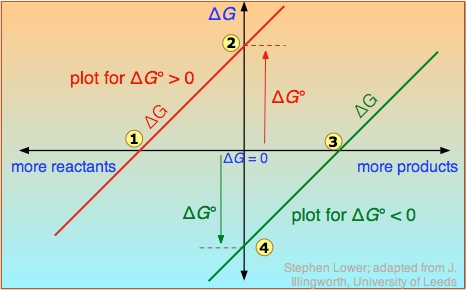ΔG vs. G°: ΔG is plotted on a vertical axis for two hypothetical reactions having opposite signs of ΔG°.

Take careful note of the following:

• The red line on the left plots the values of $\Delta \text{G}$ for the $\Delta \text{G}^{\circ}$ > 0 reaction. Notice that there are an infinite number of these values, depending on the progress of the reaction. In contrast, there is only a single value (point 2) of $\Delta \text{G}^{\circ}$, corresponding to the composition at which $\Delta \text{G}$ = 0 (point 1).
• At this point, some products have been formed, but the composition is still dominated by reactants.
• If the reaction begins at a composition to the left of point 1 on the diagram, $\Delta \text{G}$ will be negative and the composition will move to the right. Similarly, if the reaction begins with a composition to the right of point 1 on the diagram, $\Delta \text{G}$ will be positive and the composition will move to the left.
• The plot on the right is for the $\Delta \text{G}^{\circ}$ < 0 reaction, for which $\Delta \text{G}^{\circ}$is shown at point 4 on the diagram. At its equilibrium point (point 3), there are more products than reactants. If the reaction starts at a composition to the right of point 3 on the diagram, the composition will tend to move to the left. If the initial composition is to the left of point 3 on diagram, the reaction will tend to proceed to the right.
• What would happen if $\Delta \text{G}^{\circ}$ were 0? The equilibrium point of such a reaction would be at the origin, corresponding to half the reactants being converted to products.

The important principle to understand is that a negative $\Delta \text{G}^{\circ}$ does not mean that the reactants will be completely transformed into products. By the same token, a positive $\Delta \text{G}^{\circ}$ does not mean that no products are formed at all.

### Possible Values

In contrast, the composition of a chemical reaction system undergoes continual change until the equilibrium state is reached. Therefore, a single reaction can have an infinite number of $\Delta \text{G}$ values, reflecting the infinite possible compositions between the extremes of pure reactants and pure products.

In the example of a reaction $\text{A} \rightarrow \text{B}$, depicted in the following diagram, the standard free energy of the products (point 2) is smaller than that of the reactants (point 1), so the reaction will take place spontaneously. This does not mean that each mole of pure A will be converted into one mole of pure B.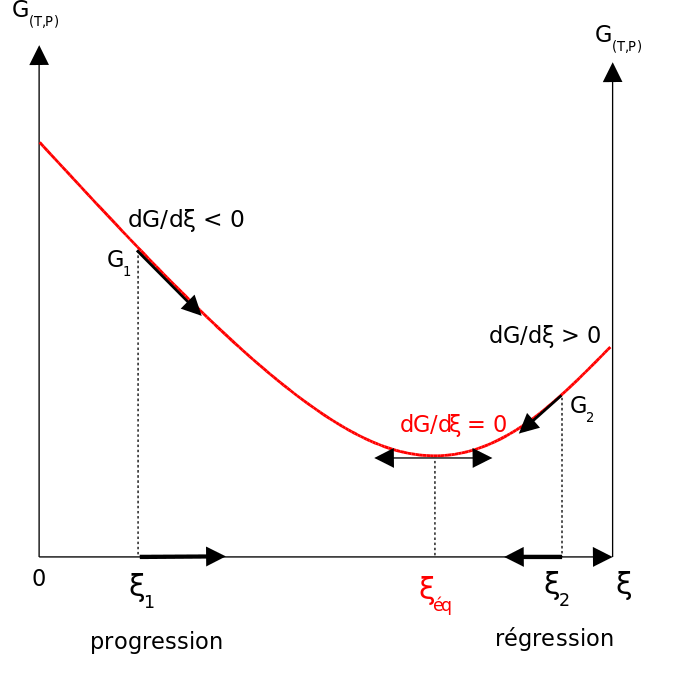Nonstandard state free energy change: Graph of $\text{G}(\text{T},\text{p}) = \text{f}(\xi)$. A single reaction can have an infinite number of $\Delta \text{G}$ values.

The physical meaning of $\Delta \text{G}$ is that it tells us how far the free energy of the system has changed from G° of the pure reactants (point 1). As the reaction proceeds to the right, the composition changes, and $\Delta \text{G}$ begins to fall. When the composition reaches point 3, $\Delta \text{G}$ reaches its minimum value, and further reaction would cause it to rise. But because free energy can only decrease but never increase, this does not happen. The composition of the system remains permanently at its equilibrium value.

## Pressure and Free Energy

Gibbs free energy is dependent on pressure.

### Learning Objectives

Discuss the relationship between free energy and pressure.

### Key Takeaways

#### Key Points

• Gibbs energy (also referred to as ∆G) is also the chemical potential that is minimized when a system reaches equilibrium at constant pressure and temperature.
• The Gibbs free energy equation is dependent on pressure.
• It is a convenient criterion of spontaneity for processes with constant pressure and temperature.

#### Key Terms

• chemical potential: In thermodynamics, chemical potential, also known as partial molar free energy, is a form of potential energy that can be absorbed or released during a chemical reaction.

Gibbs free energy measures the useful work obtainable from a thermodynamic system at a constant temperature and pressure. Just as in mechanics, where potential energy is defined as capacity to do work, similarly different potentials have different meanings. The Gibbs free energy is the maximum amount of non-expansion work that can be extracted from a closed system.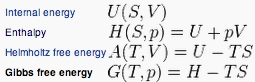Gibbs free energy equation: The Gibbs free energy equation is dependent on pressure.

When a system changes from an initial state to a final state, the Gibbs free energy (ΔG) equals the work exchanged by the system with its surroundings, minus the work of the pressure force. Gibbs energy (also referred to as ∆G) is also the chemical potential that is minimized when a system reaches equilibrium at constant pressure and temperature. As such, it is a convenient criterion of spontaneity for processes with constant pressure and temperature. Therefore, Gibbs free energy is most useful for thermochemical processes at constant temperature and pressure.

## Free Energy and Work

The Gibbs free energy is the maximum amount of non-expansion work that can be extracted from a closed system.

### Learning Objectives

Identify the relationship between Gibbs free energy and work.

### Key Takeaways

#### Key Points

• When a system changes from a well-defined initial state to a well-defined final state, the Gibbs free energy, ΔG, equals the work done/exchanged by the system with its surroundings, minus the work of the pressure forces.
• “Useful” work is most commonly electrical work (moving electric charge through a potential difference).

#### Key Terms

• work: In thermodynamics, work performed by a closed system is the energy transferred to another system that is measured by the external generalized mechanical constraints on the system.

### Gibb’s Free Energy and “Useful” Work

Gibbs energy is the maximum useful work that a system can do on its surroundings when the process occurring within the system is reversible at constant temperature and pressure. As in mechanics, where potential energy is defined as capacity to do work, different potentials have different meanings. The Gibbs free energy is the maximum amount of non-expansion work that can be extracted from a closed system. The work is done at the expense of the system’s internal energy. Energy that is not extracted as work is exchanged with the surroundings as heat.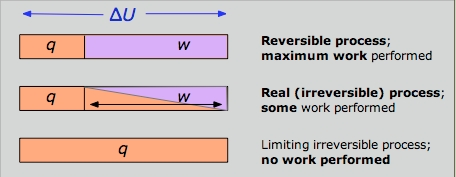Work Diagram: The reversible condition implies wmax and qmin. The impossibility of extracting all of the internal energy as work is essentially a statement of the Second Law.

The appellation “free energy” for G has led to so much confusion that many scientists now refer to it simply as the “Gibbs energy. ” The “free” part of the older name reflects the steam-engine origins of thermodynamics, with its interest in converting heat into work. ΔG is the maximum amount of energy which can be “freed” from the system to perform useful work. “Useful” in this case, refers to the work not associated with the expansion of the system. This is most commonly electrical work (moving electric charge through a potential difference), but other forms of work are also possible. For instance, examples of useful, non-expansion work in biological organisms include muscle contraction and the transmission of nerve impulses.